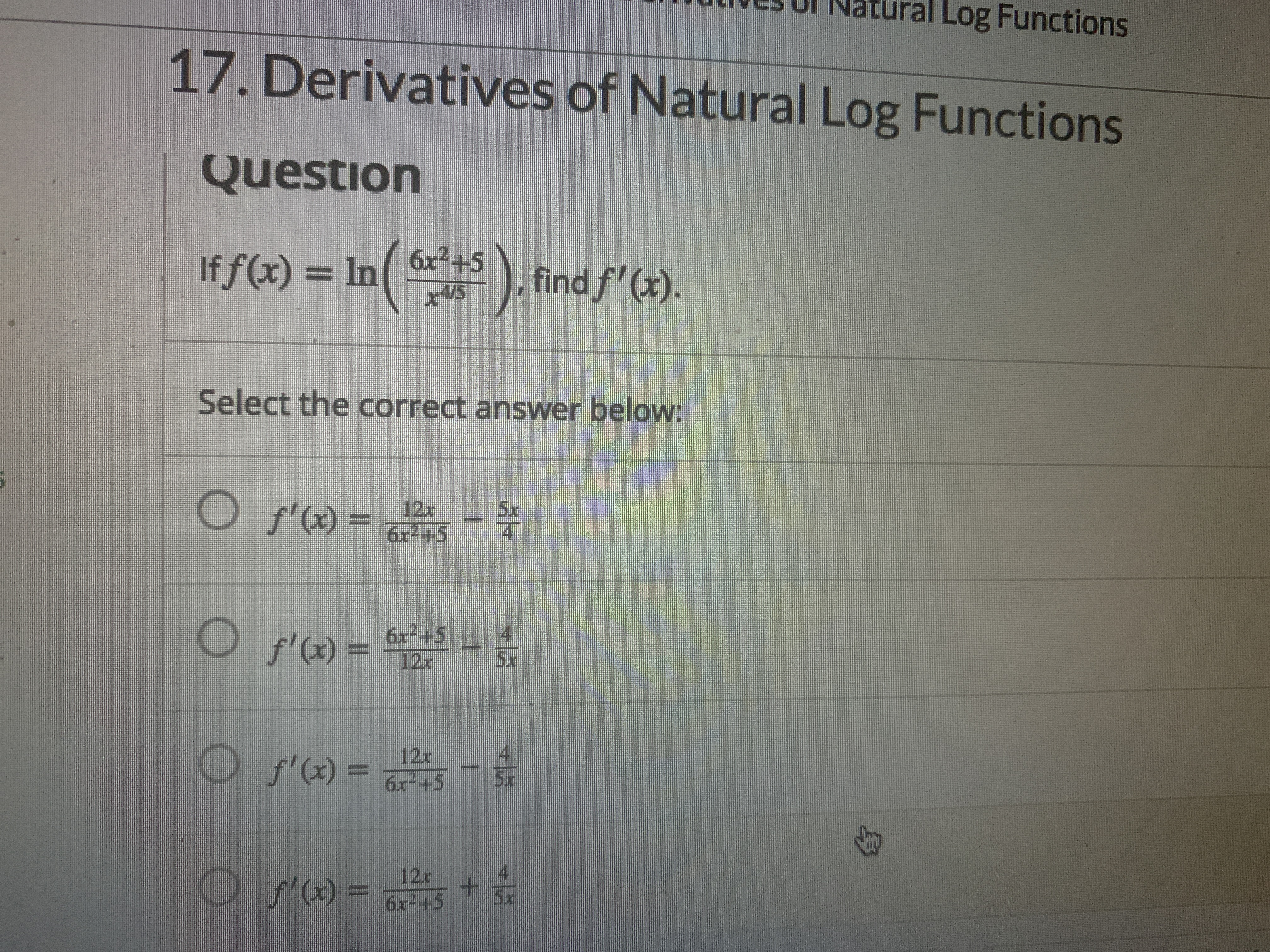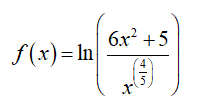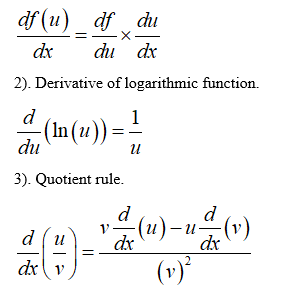# ral Log Functions17. Derivatives of Natural Log FunctionsQuestion6x²+5If f(x) = In x+5), find f'(x).4/5Select the correct answer below:f'(x) =12x62+55x6r+5f'(x) = 125xO f) =-12x6x-+55x12x6x-45+O r) =5x

Question
1 viewshelp_outlineImage Transcriptioncloseral Log Functions 17. Derivatives of Natural Log Functions Question 6x²+5 If f(x) = In x+5 ), find f'(x). 4/5 Select the correct answer below: f'(x) = 12x 62+5 5x 6r+5 f'(x) = 12 5x O f) = - 12x 6x-+5 5x 12x 6x-45 + O r) = 5x fullscreen
check_circle

Step 1

To find the derivative of the given logarithmic function.

Step 2

Given information:Step 3

Derivative formulas used:

1). Chain rule....

### Want to see the full answer?

See Solution

#### Want to see this answer and more?

Solutions are written by subject experts who are available 24/7. Questions are typically answered within 1 hour.*

See Solution
*Response times may vary by subject and question.
Tagged in